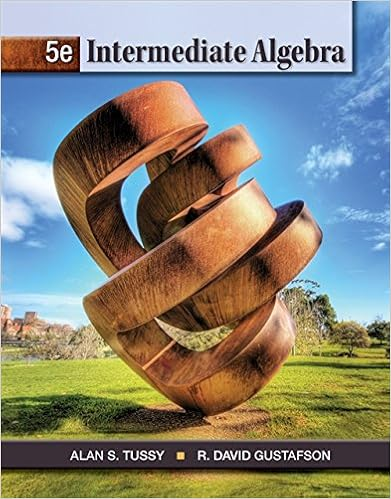Unit 5 Section 1 - Unit 5 Systems of Equations and...

• Notes
• 13

This preview shows page 1 - 3 out of 13 pages.

We have textbook solutions for you!
The document you are viewing contains questions related to this textbook.The document you are viewing contains questions related to this textbook.
Chapter 3 / Exercise 23
Intermediate Algebra
Tussy/GustafsonExpert Verified
Unit 5. Systems of Equations and Inequalities In Units 1 to 4 our study of algebra and trigonometry has been dealing with either a function of one variable or a single equation (or inequality) in two variables. However, many problems in many different fields of study give rise to two or more equations (or inequalities) in two or more variables. To solve such problems , we need to find all the solutions of a system of equations (or inequalities) . By a solution to a system of equations (or inequalities), we mean the values of the variables that satisfy each equation (or each inequality) in the system. For this last unit of Math 17 we will focus on methods for finding solutions that are common to all equations in a system. Of particular importance are the techniques involving matrices , because they are well-suited for computer implementation and can be readily applied to a system containing any number of linear equations in any number of variables. We shall also consider systems of inequalities and linear programming , a powerful mathematical tool of major importance in solving a variety of practical problems. After completing this unit, you should be able to: 1. Solve systems of nonlinear equations in two unknowns; 2. Solve systems of linear equations in two and three unknowns; 3. Solve systems of inequalities, 4. Solve linear programming problems, 5. Use systems of equations and inequalities to model and solve real-life problems. OUTLINE: 1. Systems of Equations in Two Unknowns: Substitution; Elimination 2. Systems of Linear Equations in Three Unknowns: Gaussian Elimination 3. Matrices and Determinants for Solving Linear Systems 4. Systems of Inequalities 5. Linear Programming
We have textbook solutions for you!
The document you are viewing contains questions related to this textbook.The document you are viewing contains questions related to this textbook.
Chapter 3 / Exercise 23
Intermediate Algebra
Tussy/GustafsonExpert Verified
Unit 5. Systems of Equations and Inequalities Section 1 page 2 _____________________________________________________________________________________ 5.1 Systems of Equations in Two Unknowns No doubt you learned how to solve a linear system in high school. Let us have some recall. A linear system of two equations in two variables , say x and y , is of the form 2 2 2 1 1 1 c y b x a c y b x a where a 1 , a 2 , b 1 , b 2 , c 1 , and c 2 are real numbers. Here the brace is used to indicate that the equations are to be treated simultaneously. This system can be represented graphically as a pair of lines that are intersecting, coincident, or parallel. Example 5.1.1 Examples of Systems of Equations in Two Unknowns Systems of linear equations Systems of nonlinear equations Note that in a nonlinear system, one of your equations can be linear, just not all of them. Solving Systems of Equations in Two Unknowns In general, a solution of a system of two equations in two variables is an ordered pair that makes BOTH equations true. Geometrically, it is where the graphs of the two equations intersect, what they have in common.
•••